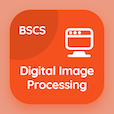Computer Science Online Courses

Digital Logic Design Quizzes

Digital Logic Design Quiz PDF - Complete

# Introduction to MSI and PLD Components Quiz MCQ Online p. 93

Practice Introduction to MSI and PLD Components quiz questions and answers PDF, introduction to msi and pld components trivia questions 93 to learn online Digital Logic Design course for online classes. MSI and PLD Components MCQ questions, introduction to msi and pld components Multiple Choice Questions (MCQ) for online college degrees. "Introduction to MSI and PLD Components Quiz" PDF eBook: clocked sequential circuits in dld, lab learning in dld, introduction to msi and pld components test prep for computer software engineer online degree.

"PAL stands for" MCQ PDF: programmable advanced logic, portable array logic, programmable array logic, and portable advanced logic for computer software engineer. Solve msi and pld components questions and answers to improve problem solving skills to learn online certificate courses.

## Trivia Quiz on Introduction to MSI & PLD Components MCQs

MCQ: PAL stands for

Portable Array Logic
Programmable Array Logic

MCQ: The input of the Binary Coded Decimal (BCD) counter is connected to the

gate
voltage
pulsar
current

MCQ: The next stage of the D flip-flop is dependent on

state diagram
present state
input state
D state

MCQ: Ripple counter is a type of

SSI counters
LSI counters
MSI counters
VLSI counters

MCQ: Medium Scale Integration (MSI) counter comes in two categories, one is Ripple counter and second is

ripple fashion
serial ripple
parallel ripple
Synchronous counter

### More Quizzes from Digital Logic Design Course